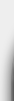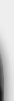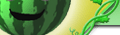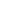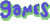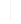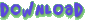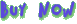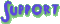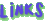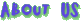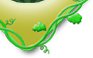# Peepers - Free Hints and Solutions

The following table lists hints and best solutions for all levels in Peepers. Each solution lists the directions that the frog should hop (U=up, D=down, L=left, R=right).

If multiple frogs are in a level, the frogs are given numbers and identified in the level by their column and row position (i.e. a frog at (1,5) is in the lower left-hand corner). The solutions for multiple frogs then show the frog number followed by a direction to hop (e.g. 1U or 2D).

 Level 1 Hint Solution Level 2 Hint Solution Level 3 Hint Solution Level 4 Hint Solution Level 5 Hint Solution Level 6 Hint Solution Level 7 Hint Solution Level 8 Hint Solution Level 9 Hint Solution Level 10 Hint Solution Level 11 Hint Solution Level 12 Hint Solution Level 13 Hint Solution Level 14 Hint Solution Level 15 Hint Solution Level 16 Hint Solution Level 17 Hint Solution Level 18 Hint Solution Level 19 Hint Solution Level 20 Hint Solution Level 21 Hint Solution Level 22 Hint Solution Level 23 Hint Solution Level 24 Hint Solution Level 25 Hint Solution Level 26 Hint Solution Level 27 Hint Solution Level 28 Hint Solution Level 29 Hint Solution Level 30 Hint Solution Level 31 Hint Solution Level 32 Hint Solution Level 33 Hint Solution Level 34 Hint Solution Level 35 Hint Solution Level 36 Hint Solution Level 37 Hint Solution Level 38 Hint Solution Level 39 Hint Solution Level 40 Hint Solution Level 41 Hint Solution Level 42 Hint Solution Level 43 Hint Solution Level 44 Hint Solution Level 45 Hint Solution Level 46 Hint Solution Level 47 Hint Solution Level 48 Hint Solution Level 49 Hint Solution Level 50 Hint Solution Level 51 Hint Solution Level 52 Hint Solution Level 53 Hint Solution Level 54 Hint Solution Level 55 Hint Solution Level 56 Hint Solution Level 57 Hint Solution Level 58 Hint Solution Level 59 Hint Solution Level 60 Hint Solution Level 61 Hint Solution Level 62 Hint Solution Level 63 Hint Solution Level 64 Hint Solution Level 65 Hint Solution Level 66 Hint Solution Level 67 Hint Solution Level 68 Hint Solution Level 69 Hint Solution Level 70 Hint Solution Level 71 Hint Solution Level 72 Hint Solution Level 73 Hint Solution Level 74 Hint Solution Level 75 Hint Solution Level 76 Hint Solution Level 77 Hint Solution Level 78 Hint Solution Level 79 Hint Solution Level 80 Hint Solution Level 81 Hint Solution Level 82 Hint Solution Level 83 Hint Solution Level 84 Hint Solution Level 85 Hint Solution Level 86 Hint Solution Level 87 Hint Solution Level 88 Hint Solution Level 89 Hint Solution Level 90 Hint Solution Level 91 Hint Solution Level 92 Hint Solution Level 93 Hint Solution Level 94 Hint Solution Level 95 Hint Solution Level 96 Hint Solution Level 97 Hint Solution Level 98 Hint Solution Level 99 Hint Solution Level 100 Hint Solution Bonus Level 1 Hint Solution Bonus Level 2 Hint Solution Bonus Level 3 Hint Solution Bonus Level 4 Hint Solution Bonus Level 5 Hint Solution Bonus Level 6 Hint Solution Bonus Level 7 Hint Solution Bonus Level 8 Hint Solution Bonus Level 9 Hint Solution Bonus Level 10 Hint Solution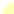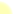Our Games:     TangleBee     Peepers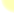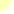Newsletter: Join our free mailing list to find out when our next game is released, plus special offers, tips and tricks, and important news. Your privacy is important to us.Our Guarantee: If you are not satisfied with the purchase of any of our games, return the game within 60 days for a full refund!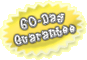Free Hints and Solutions